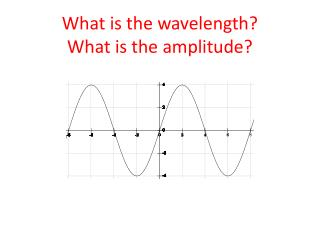# What is the wavelength? What is the amplitude? - PowerPoint PPT PresentationDownload PresentationWhat is the wavelength? What is the amplitude?

Download Presentation## What is the wavelength? What is the amplitude?

- - - - - - - - - - - - - - - - - - - - - - - - - - - E N D - - - - - - - - - - - - - - - - - - - - - - - - - - -
##### Presentation Transcript

1. What is the wavelength?What is the amplitude?

2. What is the wavelength?What is the amplitude?

3. After exercising, your heart beats about 2 times per second.What is the frequency?What is the period?

4. What is the velocity of a water wave if the frequency is 5 Hz and the wavelength is 12 m?

5. A wave’s period is 0.25 seconds. What’s its frequency?What’s its speed if the wavelength is 2 meters?

6. A pendulum completes one cycle in 4 seconds.What is the frequency?What is the period?

7. After exercising, your heart beats about 2 times per second.What is the frequency?What is the period?

8. A sound wave has a velocity of 343 m/s. Calculate its frequency if the wavelength is 175 m

9. WIBC broadcasts at 93.1 MHz. That’s 93,100,000 Hz. The speed of radio waves is 300,000,000 m/s. What is the wavelength of the WIBC broadcast?

10. What is the speed of a wave if its wavelength is 20 meters and its frequency is 50 Hz?

11. What is the velocity of a water wave if the frequency is 5 Hz and the wavelength is 12 m?

12. You’re playing jump rope. You make your arm go up and down 3 times per second. The distance between you and your partner (half of the wavelength) is 4 meters. What is the wave speed?

13. What is the wavelength of a water wave if its frequency is 5 Hz and its velocity is 35 m/s?

14. You’re on a boat. The crest of the incoming waves pass you every 10 seconds. The waves travel at5 m/s. What is they’re wavelength